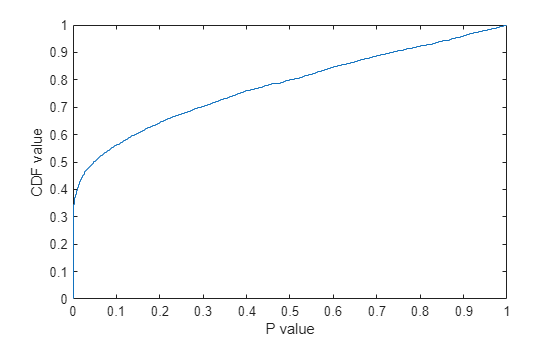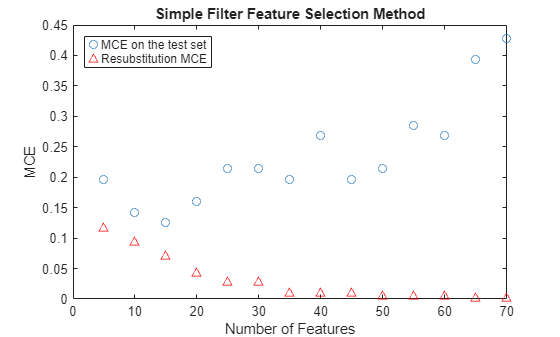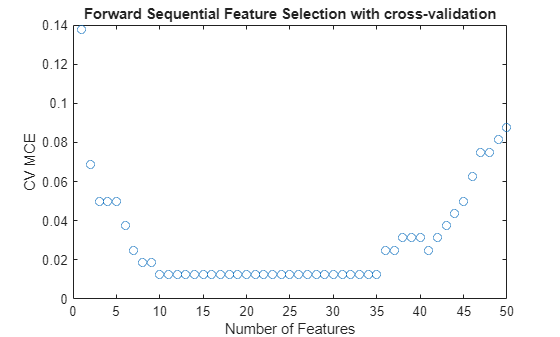# 选择用于高维数据分类的特征

### 加载数据

```load ovariancancer; whos```
``` Name Size Bytes Class Attributes grp 216x1 25056 cell obs 216x4000 3456000 single ```

### 将数据分成训练集和测试集

`rng(8000,'twister');`

`holdoutCVP = cvpartition(grp,'holdout',56)`
```holdoutCVP = Hold-out cross validation partition NumObservations: 216 NumTestSets: 1 TrainSize: 160 TestSize: 56 ```
```dataTrain = obs(holdoutCVP.training,:); grpTrain = grp(holdoutCVP.training);```

### 使用全部特征对数据进行分类存在的问题

```try yhat = classify(obs(test(holdoutCVP),:), dataTrain, grpTrain,'quadratic'); catch ME display(ME.message); end```
```The covariance matrix of each group in TRAINING must be positive definite. ```

### 使用简单的筛选器方法选择特征

```dataTrainG1 = dataTrain(grp2idx(grpTrain)==1,:); dataTrainG2 = dataTrain(grp2idx(grpTrain)==2,:); [h,p,ci,stat] = ttest2(dataTrainG1,dataTrainG2,'Vartype','unequal');```

```ecdf(p); xlabel('P value'); ylabel('CDF value')``````[~,featureIdxSortbyP] = sort(p,2); % sort the features testMCE = zeros(1,14); resubMCE = zeros(1,14); nfs = 5:5:70; classf = @(xtrain,ytrain,xtest,ytest) ... sum(~strcmp(ytest,classify(xtest,xtrain,ytrain,'quadratic'))); resubCVP = cvpartition(length(grp),'resubstitution') ```
```resubCVP = Resubstitution (no partition of data) NumObservations: 216 NumTestSets: 1 TrainSize: 216 TestSize: 216 ```
```for i = 1:14 fs = featureIdxSortbyP(1:nfs(i)); testMCE(i) = crossval(classf,obs(:,fs),grp,'partition',holdoutCVP)... /holdoutCVP.TestSize; resubMCE(i) = crossval(classf,obs(:,fs),grp,'partition',resubCVP)/... resubCVP.TestSize; end plot(nfs, testMCE,'o',nfs,resubMCE,'r^'); xlabel('Number of Features'); ylabel('MCE'); legend({'MCE on the test set' 'Resubstitution MCE'},'location','NW'); title('Simple Filter Feature Selection Method');``````% function err = classf(xtrain,ytrain,xtest,ytest) % yfit = classify(xtest,xtrain,ytrain,'quadratic'); % err = sum(~strcmp(ytest,yfit));```

`testMCE(3)`
```ans = 0.1250 ```

`featureIdxSortbyP(1:15)`
```ans = 1×15 2814 2813 2721 2720 2452 2645 2644 2642 2650 2643 2731 2638 2730 2637 2398 ```

### 应用序列特征选择

`corr(dataTrain(:,featureIdxSortbyP(1)),dataTrain(:,featureIdxSortbyP(2)))`
```ans = single 0.9447 ```

`tenfoldCVP = cvpartition(grpTrain,'kfold',10)`
```tenfoldCVP = K-fold cross validation partition NumObservations: 160 NumTestSets: 10 TrainSize: 144 144 144 144 144 144 144 144 144 144 TestSize: 16 16 16 16 16 16 16 16 16 16 ```

`fs1 = featureIdxSortbyP(1:150);`

` fsLocal = sequentialfs(classf,dataTrain(:,fs1),grpTrain,'cv',tenfoldCVP);`

`fs1(fsLocal)`
```ans = 1×3 2337 864 3288 ```

```testMCELocal = crossval(classf,obs(:,fs1(fsLocal)),grp,'partition',... holdoutCVP)/holdoutCVP.TestSize```
```testMCELocal = 0.0714 ```

```[fsCVfor50,historyCV] = sequentialfs(classf,dataTrain(:,fs1),grpTrain,... 'cv',tenfoldCVP,'Nf',50); plot(historyCV.Crit,'o'); xlabel('Number of Features'); ylabel('CV MCE'); title('Forward Sequential Feature Selection with cross-validation');````fsCVfor10 = fs1(historyCV.In(10,:))`
```fsCVfor10 = 1×10 2814 2721 2720 2452 2650 2731 2337 2658 864 3288 ```

```[orderlist,ignore] = find( [historyCV.In(1,:); diff(historyCV.In(1:10,:) )]' ); fs1(orderlist)```
```ans = 1×10 2337 864 3288 2721 2814 2658 2452 2731 2650 2720 ```

```testMCECVfor10 = crossval(classf,obs(:,fsCVfor10),grp,'partition',... holdoutCVP)/holdoutCVP.TestSize```
```testMCECVfor10 = 0.0357 ```

```[fsResubfor50,historyResub] = sequentialfs(classf,dataTrain(:,fs1),... grpTrain,'cv','resubstitution','Nf',50); plot(1:50, historyCV.Crit,'bo',1:50, historyResub.Crit,'r^'); xlabel('Number of Features'); ylabel('MCE'); legend({'10-fold CV MCE' 'Resubstitution MCE'},'location','NE');``````fsResubfor16 = fs1(historyResub.In(16,:)); testMCEResubfor16 = crossval(classf,obs(:,fsResubfor16),grp,'partition',... holdoutCVP)/holdoutCVP.TestSize```
```testMCEResubfor16 = 0.0714 ```